# contour

Contour plot of matrix

•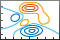## Syntax

``contour(Z)``
``contour(X,Y,Z)``
``contour(___,levels)``
``contour(___,LineSpec)``
``contour(___,Name,Value)``
``contour(ax,___)``
``M = contour(___)``
``[M,c] = contour(___)``

## Description

example

````contour(Z)` creates a contour plot containing the isolines of matrix `Z`, where `Z` contains height values on the x-y plane. MATLAB® automatically selects the contour lines to display. The column and row indices of `Z` are the x and y coordinates in the plane, respectively.```

example

````contour(X,Y,Z)` specifies the x and y coordinates for the values in `Z`.```

example

````contour(___,levels)` specifies the contour lines to display as the last argument in any of the previous syntaxes. Specify `levels` as a scalar value `n` to display the contour lines at `n` automatically chosen levels (heights). To draw the contour lines at specific heights, specify `levels` as a vector of monotonically increasing values. To draw the contours at one height (`k`), specify `levels` as a two-element row vector `[k k]`.```

example

````contour(___,LineSpec)` specifies the style and color of the contour lines.```

example

````contour(___,Name,Value)` specifies additional options for the contour plot using one or more name-value pair arguments. Specify the options after all other input arguments. For a list of properties, see Contour Properties.```
````contour(ax,___)` displays the contour plot in the target axes. Specify the axes as the first argument in any of the previous syntaxes.```
````M = contour(___)` returns the contour matrix `M`, which contains the (x, y) coordinates of the vertices at each level.```

example

````[M,c] = contour(___)` returns the contour matrix and the contour object `c`. Use `c` to set properties after displaying the contour plot.```

## Examples

collapse all

Create matrices `X` and `Y`, that define a grid in the x-y plane. Define matrix `Z` as the heights above that grid. Then plot the contours of `Z`.

```x = linspace(-2*pi,2*pi); y = linspace(0,4*pi); [X,Y] = meshgrid(x,y); Z = sin(X)+cos(Y); contour(X,Y,Z)```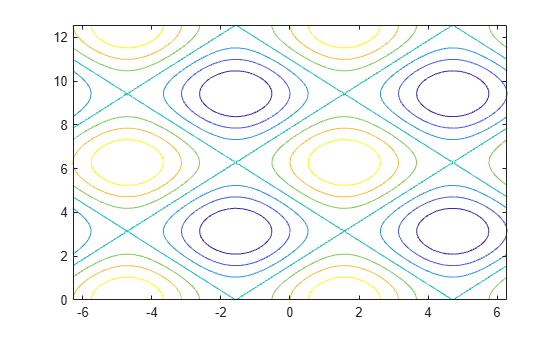Define `Z` as a function of `X` and `Y`. In this case, call the `peaks` function to create `X`, `Y`, and `Z`. Then plot 20 contours of `Z`.

```[X,Y,Z] = peaks; contour(X,Y,Z,20)```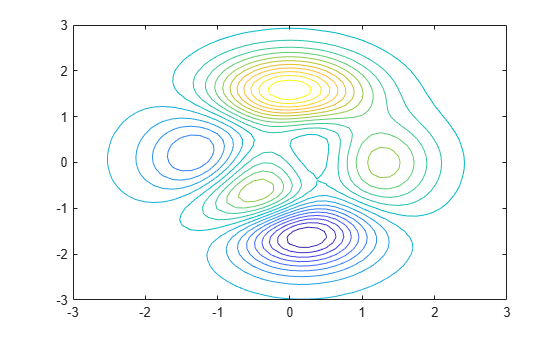Display the contours of the `peaks` function at `Z = 1`.

```[X,Y,Z] = peaks; v = [1,1]; contour(X,Y,Z,v)```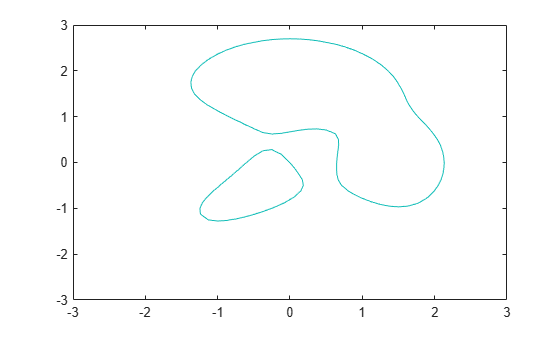Create a contour plot of the `peaks` function, and specify the dashed line style.

```[X,Y,Z] = peaks; contour(X,Y,Z,'--')```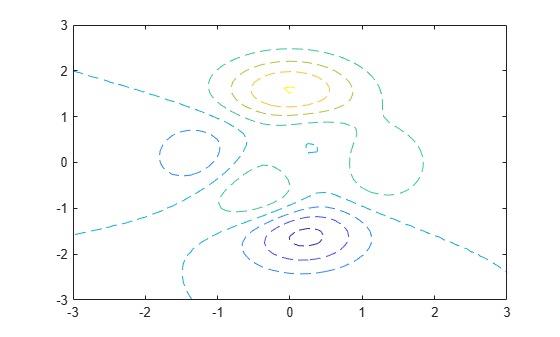Define `Z` as a function of two variables, `X` and `Y`. Then create a contour plot of that function, and display the labels by setting the `ShowText` property to `'on'`.

```x = -2:0.2:2; y = -2:0.2:3; [X,Y] = meshgrid(x,y); Z = X.*exp(-X.^2-Y.^2); contour(X,Y,Z,'ShowText','on')```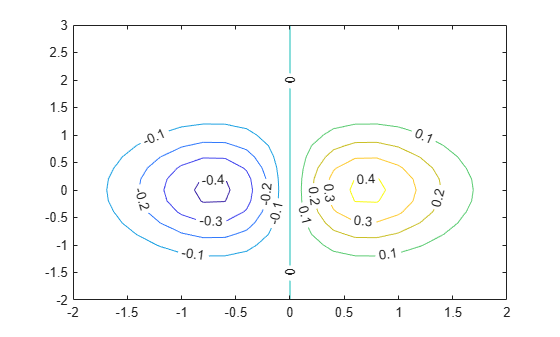Since R2022b

Create a contour plot that displays labels with one digit after the decimal point followed by the letter `m`.

`contour(peaks,[-4 0 2],"ShowText",true,"LabelFormat","%0.1f m")`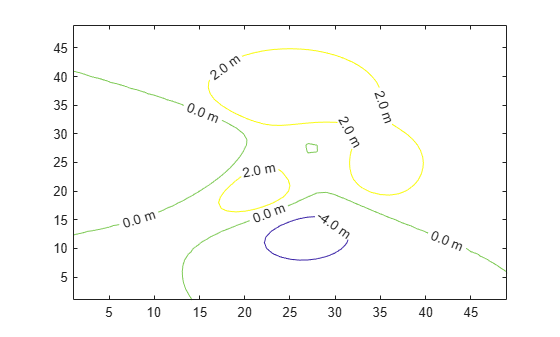Since R2022b

You can specify a function to format the labels when you need to calculate values. For example, you can define a function to calculate the label values in different units.

Define this function in a program file called `mylabelfun.m`. The function converts the input from meters to feet and returns a string vector containing each value in meters with the equivalent value in feet in parentheses.

```function labels = mylabelfun(vals) feetPerMeter = 3.28084; feet = round(vals.*feetPerMeter); labels = vals + " m (" + feet + " ft)"; labels(vals == 0) = "0 m"; end ```

Next, create a contour plot and specify the `LabelFormat` property as a handle to `mylabelfun`.

```contour(peaks,[-4 0 2],"ShowText",true,"LabelFormat",@mylabelfun) ```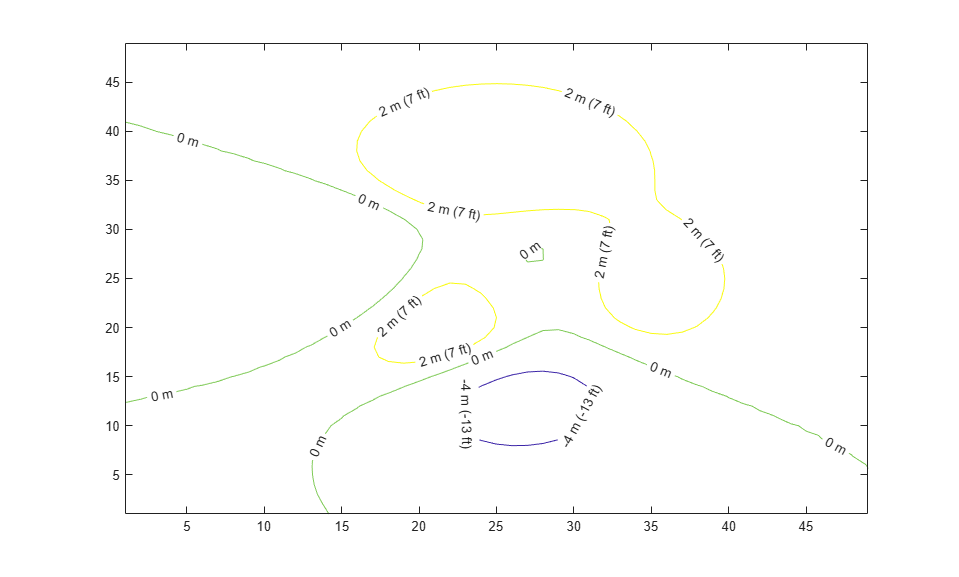Create a contour plot of the `peaks` function. Make the contour lines thicker by setting the `LineWidth` property to `3`.

```Z = peaks; [M,c] = contour(Z); c.LineWidth = 3;```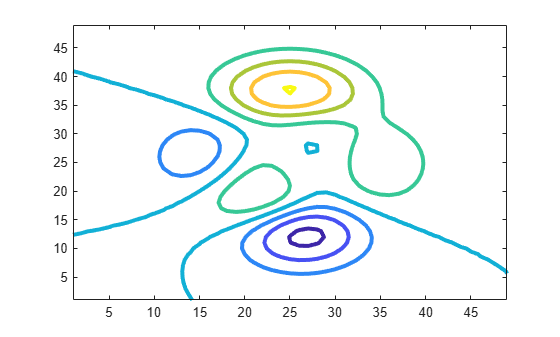Insert `NaN` values wherever there are discontinuities on a surface. The `contour` function does not draw contour lines in those regions.

Define matrix `Z` as a sampling of the `peaks` function. Replace all values in column `26` with `NaN` values. Then plot the contours of the modified `Z` matrix.

```Z = peaks; Z(:,26) = NaN; contour(Z)```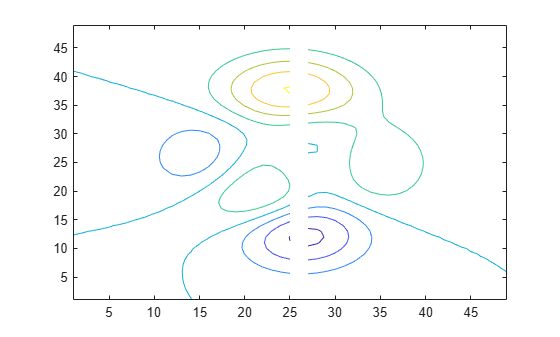## Input Arguments

collapse all

x-coordinates, specified as a matrix the same size as `Z`, or as a vector with length `n`, where `[m,n] = size(Z)`. The default value of `X` is the vector `(1:n)`.

When `X` is a matrix, the values must be strictly increasing or decreasing along one dimension and remain constant along the other dimension. The dimension that varies must be the opposite of the dimension that varies in `Y`. You can use the `meshgrid` function to create `X` and `Y` matrices.

When `X` is a vector, the values must be strictly increasing or decreasing.

Example: `X = 1:10`

Example: `X = [1 2 3; 1 2 3; 1 2 3]`

Example: `[X,Y] = meshgrid(1:10)`

The `XData` property of the `Contour` object stores the x-coordinates.

Data Types: `single` | `double` | `int8` | `int16` | `int32` | `int64` | `uint8` | `uint16` | `uint32` | `uint64`

y-coordinates, specified as a matrix the same size as `Z`, or as a vector with length `m`, where `[m,n] = size(Z)`. The default value of `Y` is the vector `(1:m)`.

When `Y` is a matrix, the values must be strictly increasing or decreasing along one dimension and remain constant along the other dimension. The dimension that varies must be the opposite of the dimension that varies in `X`. You can use the `meshgrid` function to create the `X` and `Y` matrices.

When `Y` is a vector, the values must be strictly increasing or decreasing.

Example: `Y = 1:10`

Example: `Y = [1 1 1; 2 2 2; 3 3 3]`

Example: `[X,Y] = meshgrid(1:10)`

The `YData` property of the `Contour` object stores the y-coordinates.

Data Types: `single` | `double` | `int8` | `int16` | `int32` | `int64` | `uint8` | `uint16` | `uint32` | `uint64`

z-coordinates, specified as a matrix. This matrix must have at least two rows and two columns, and it must contain at least two different values.

Example: `Z = peaks(20)`

The `ZData` property of the `Contour` object stores the z-coordinates.

Data Types: `single` | `double` | `int8` | `int16` | `int32` | `int64` | `uint8` | `uint16` | `uint32` | `uint64`

Contour levels, specified as a scalar whole number or a vector. Use this argument to control the number and location of the contour lines. When you do not specify the levels, the `contour` function chooses the levels automatically.

• To draw contour lines at n automatically chosen heights, specify levels as the scalar value n.

• To draw the contour lines at specific heights, specify `levels` as a vector of monotonically increasing values.

• To draw contour lines at a single height `k`, specify `levels` as a two-element row vector ```[k k]```.

Example: `contour(peaks,10)` draws contour lines at 10 automatically chosen heights on the `peaks` function.

Example: `contour(peaks,[-4 0 4])` draws contour lines at 3 specific heights on the `peaks` function: `-4`, `0`, and `4`.

Example: `contour(peaks,[3 3])` draws contour lines to show where the height of the `peaks` function is `3`.

Data Types: `single` | `double` | `int8` | `int16` | `int32` | `int64` | `uint8` | `uint16` | `uint32` | `uint64`

Line style and color, specified as a character vector or string scalar containing characters and symbols. The characters and symbols can appear in any order. You can specify the line style, line color, or both. Marker symbols such as `'o'` are ignored.

Example: `'--g'` is a green dashed line.

Line StyleDescriptionResulting Line
`"-"`Solid line`"--"`Dashed line`":"`Dotted line`"-."`Dash-dotted lineColor NameShort NameAppearance
`'red'``'r'``'green'``'g'``'blue'``'b'``'cyan'` `'c'``'magenta'``'m'``'yellow'``'y'``'black'``'k'``'white'``'w'`Target axes, specified as an `Axes` object. If you do not specify the axes, then `contour` plots into the current axes.

### Name-Value Arguments

Specify optional pairs of arguments as `Name1=Value1,...,NameN=ValueN`, where `Name` is the argument name and `Value` is the corresponding value. Name-value arguments must appear after other arguments, but the order of the pairs does not matter.

Before R2021a, use commas to separate each name and value, and enclose `Name` in quotes.

Example: `contour(Z,'ShowText','on')` displays the contour line labels.

Note

The properties listed here are only a subset. For a complete list, see Contour Properties.

Contour line labels, specified as `'on'` or `'off'`, or as numeric or logical `1` (`true`) or `0` (`false`). A value of `'on'` is equivalent to `true`, and `'off'` is equivalent to `false`. Thus, you can use the value of this property as a logical value. The value is stored as an on/off logical value of type `matlab.lang.OnOffSwitchState`.

• `'on'` — Display the height values along the contour lines.

• `'off'` — Do not label the contour lines.

Contour line width, specified as a positive value in points. One point equals 1/72 inch.

Label spacing along the contour lines, specified as a scalar value in points, where one point is 1/72 inch. Use this property to control the number of contour labels along the contour lines. Smaller values produce more labels.

You must set the `ShowText` property to `'on'` for the `LabelSpacing` property to have an effect.

If you use the `clabel` function to display the labels, then the `LabelSpacing` property has no effect and the plot displays one label per line.

## Output Arguments

collapse all

Contour matrix, returned as a two-row matrix of following form.

```Z1, x1,1, x1,2, ..., x1,N1, Z2, x2,1, x2,2, ..., x2,N2, Z3, ... N1, y1,1, y1,2, ..., y1,N1, N2, y2,1, y2,2, ..., y2,N2, N3, ...```

The columns of the matrix define the contour lines. Each contour line starts with a column containing Z and N values:

• Zi — The height of the ith contour line

• Ni — The number of vertices in the ith contour line

• (xij, yij) — The coordinates of the vertices for the ith contour line, where j ranges from 1 to Ni

`Contour` object. Use this object to set properties after displaying the contour plot.

## Version History

Introduced before R2006a